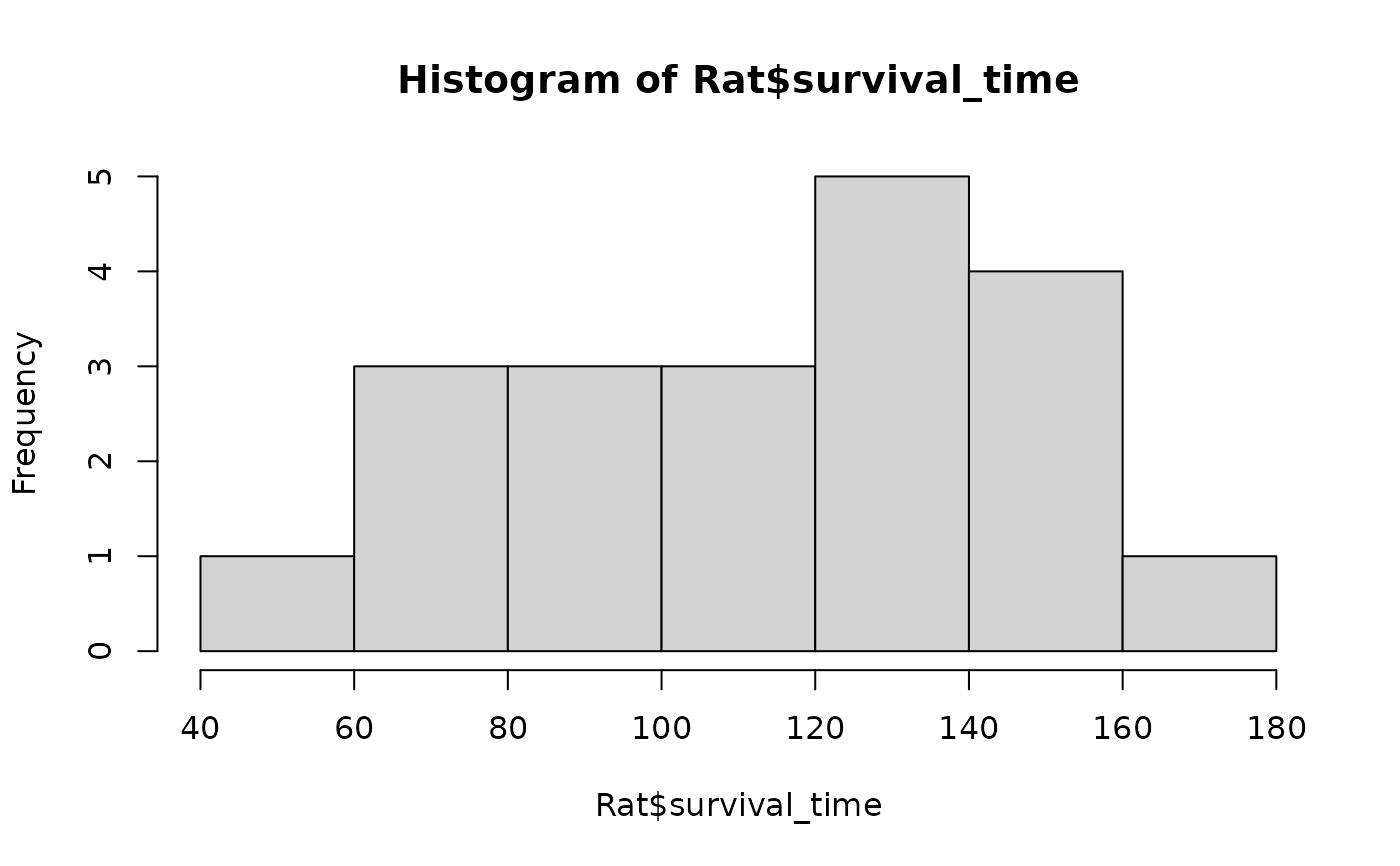Data for Exercise 1.52, 1.76, 5.62, and 6.44

Rat

## Format

A data frame/tibble with 20 observations on one variable

survival_time

survival time in weeks for rats exposed to a high level of radiation

## Source

J. Lawless, Statistical Models and Methods for Lifetime Data (New York: Wiley, 1982).

## Examples


hist(Rat$survival_time)qqnorm(Rat$survival_time)
qqline(Rat$survival_time)summary(Rat$survival_time)
#>    Min. 1st Qu.  Median    Mean 3rd Qu.    Max.
#>   40.00   86.75  119.00  113.45  140.75  165.00
t.test(Rat$survival_time) #> #> One Sample t-test #> #> data: Rat$survival_time
#> t = 14.176, df = 19, p-value = 1.48e-11
#> alternative hypothesis: true mean is not equal to 0
#> 95 percent confidence interval:
#>   96.69997 130.20003
#> sample estimates:
#> mean of x
#>    113.45
#>
t.test(Rat$survival_time, mu = 100, alternative = "greater") #> #> One Sample t-test #> #> data: Rat$survival_time
#> t = 1.6807, df = 19, p-value = 0.0546
#> alternative hypothesis: true mean is greater than 100
#> 95 percent confidence interval:
#>  99.61212      Inf
#> sample estimates:
#> mean of x
#>    113.45
#>